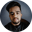Related Tags

python
recursion
communitycreator

# How to print 1 to n using recursion in PythonShubham Singh Kshatriya

### Overview

Recursion is a very common concept in all programming languages. It means a function can call itself repeatedly until it finds a terminating condition.

In this shot, we’ll see how to print 1 to n using recursion.

### Algorithm

Let’s go through the approach to solving this problem first.

• We define a function that accepts a number n.
• We recursively call the function and print the value of n, until the value of n is greater than 0.

### Example

The code snippet below demonstrates how to print 1 to n using recursion.

# python3 program to print 1 to n using recursion

def printNumber(n):
# check if n is greater than 0
if n > 0:
# recursively call the printNumber function
printNumber(n - 1)
# print n
print(n, end = ' ')

# declare the value of n
n = 50
# call the printNumber function
printNumber(n)
Printing 1 to n using recursion

### Explanation

• Line 3: We define a function printNumber() that accepts a parameter n.
• Line 5: We check if the value of n is greater than 0.
• Lines 7–9: If the above condition is true, we recursively call the function printNumber() with n - 1, and print the value of n on the console. We use the end attribute in print() function to format the output.
• Line 12: We declare and initialize a variable n.
• Line 14: We call the function printNumber() and pass n as an argument.

### Output

In the output, we can see all the numbers between 1 and n are separated by spaces.

RELATED TAGS

python
recursion
communitycreator

CONTRIBUTORShubham Singh Kshatriya
RELATED COURSES

View all Courses

Keep Exploring

Learn in-demand tech skills in half the time